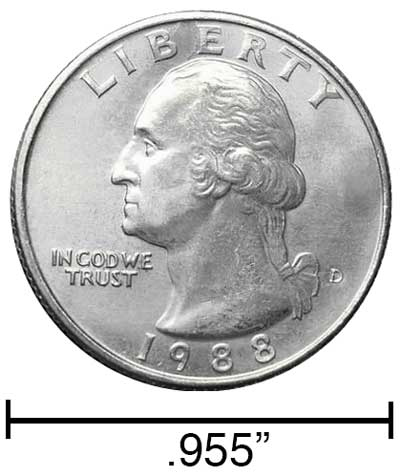# Inches to Kilometers Conversion

Enter the length in inches below to get the value converted to kilometers.

/
Results in Kilometers:1 in = 2.54E-5 km

## How to Convert Inches to KilometersTo convert an inch measurement to a kilometer measurement, multiply the length by the conversion ratio.

Since one inch is equal to 2.54E-5 kilometers, you can use this simple formula to convert:

kilometers = inches × 2.54E-5

The length in kilometers is equal to the inches multiplied by 2.54E-5.

For example, here's how to convert 50,000 inches to kilometers using the formula above.
50,000" = (50,000 × 2.54E-5) = 1.27 km

Our inch fraction calculator can add inches and kilometers together, and it also automatically converts the results to US customary, imperial, and SI metric values.

## Inches

An inch is a unit of linear length measure equal to 1/12 of a foot or 1/36 of a yard. Because the international yard is legally defined to be equal to exactly 0.9144 meters, one inch is equal to 2.54 centimeters.

The inch is a US customary and imperial unit of length. Inches can be abbreviated as in; for example, 1 inch can be written as 1 in.

Inches can also be denoted using the symbol, otherwise known as a double-prime. Often a double-quote (") is used instead of a double-prime for convenience. A double-prime is commonly used to express 1 in as 1″.The standard ruler has 12", and is a common measuring tool for measuring inches. They are also often measured using tape measures, which commonly come in lengths from 6' - 35'. Other types of measuring devices include scales, calipers, measuring wheels, micrometers, yardsticks, and even lasers.

## Kilometers

One kilometer is equal to 1,000 meters, which are defined as the distance light travels in a vacuum in a 1/299,792,458 second time interval.

The kilometer, or kilometre, is a multiple of the meter, which is the SI base unit for length. In the metric system, "kilo" is the prefix for 103. Kilometers can be abbreviated as km; for example, 1 kilometer can be written as 1 km.

We recommend using a ruler or tape measure for measuring length, which can be found at a local retailer or home center. Rulers are available in imperial, metric, or combination with both values, so make sure you get the correct type for your needs.

Need a ruler? Try our free downloadable and printable rulers, which include both imperial and metric measurements.

## Inch to Kilometer Conversion Table

Inch measurements converted to kilometers
Inches Kilometers
1" 0.0000254 km
2" 0.0000508 km
3" 0.0000762 km
4" 0.000102 km
5" 0.000127 km
6" 0.000152 km
7" 0.000178 km
8" 0.000203 km
9" 0.000229 km
10" 0.000254 km
100" 0.00254 km
1,000" 0.0254 km
10,000" 0.254 km
100,000" 2.54 km

## References

1. National Institute of Standards and Technology, Checking the Net Contents of Packaged Goods, Handbook 133 - 2019 Edition, https://nvlpubs.nist.gov/nistpubs/hb/2019/NIST.HB.133-2019.pdf
2. Ambler Thompson and Barry N. Taylor, Guide for the Use of the International System of Units (SI), National Institute of Standards and Technology, https://physics.nist.gov/cuu/pdf/sp811.pdf